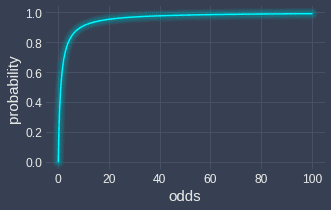search
Search
Map of Data Science
search toc
Thanks for the thanks!
close
account_circle
Profile
exit_to_app
Sign out
search
keyboard_voice
close
Searching Tips
Search for a recipe:
"Creating a table in MySQL"
Search for an API documentation: "@append"
Search for code: "!dataframe"
Apply a tag filter: "#python"
Useful Shortcuts
/ to open search panel
Esc to close search panel
to navigate between search results
d to clear all current filters
Enter to expand content previewDoc SearchCode Search BetaSORRY NOTHING FOUND!
mic
Start speaking...Voice search is only supported in Safari and Chrome.
Shrink
Navigate to
1. Basics of statistics
2. Basics of probability theory
3. Random variables
4. Point estimation
5. Discrete probability distributions
check_circle
Mark as learned
thumb_up
0
thumb_down
0
chat_bubble_outline
0
auto_stories new
settings

# Comprehensive guide on odds and odds ratio in statistics and probability

schedule Mar 5, 2023
Last updated
local_offer
Probability and Statistics
Tags
map
Check out the interactive map of data science

# What are odds?

Odds are defined by the probability that an event will occur divided by the probability that an event will not occur:

$$\begin{equation}\label{eq:VHPwuNgaRATtQCAveQl} \mathrm{odds}=\frac{\mathbb{P}(\mathrm{occurring})}{\mathbb{P}(\text{not occurring})} =\frac{p}{1-p} \end{equation}$$

Odds are related to the concept of probability, but are expressed differently. As we shall demonstrate later, we can easily interchange between odds and probability.

# Example of calculating odds when tossing a coin

Suppose we flipped a fair coin. The odds of getting a heads are:

\begin{align*} \mathrm{odds}(\mathrm{heads}) &=\frac{\mathbb{P}(\mathrm{heads})}{\mathbb{P}(\mathrm{tails})} \\&=\frac{0.5}{0.5} \\&=1 \end{align*}

Here, note the following:

• we can write this as $1:1$ (one to one) odds of getting a heads

• we can interpret this as the probability of getting a heads is equal to the probability of getting a tails

• another interpretation is that for every 2 flips, we should expect one heads and one tails

• if we get an odds value equal to $1$, this implies that the probability that the event will occur is $50\%$.

# Example of calculating odds when rolling a die

Suppose we rolled a fair die. The odds of getting a 5 or 6 are:

\begin{align*} \mathrm{odds}(5\text{ or }6) &=\frac{\mathbb{P}(5\text{ or }6)}{\mathbb{P}(1\text{ or }2\text{ or }3\text{ or }4)}\\ &=\frac{0.333}{0.666}\\ &=0.5 \end{align*}

Note the following:

• we can write this as $1:2$ (one to two) odds of rolling a 5 or 6.

• we can interpret this as the probability of flipping a 5 or 6 is half of flipping a 1, 2, 3 or 4.

• another interpretation is that for every 3 rolls, we should expect 1 success (5 or 6) and 2 failures (1,2,3 or 4).

• if we get an odds value less than 1, this implies that the probability of the event occurring is less than $50\%$.

# Converting between probability and odds

## Converting from probability to odds

Suppose event $A$ occurs at a probability of $0.8$:

$$\mathbb{P}(A)=0.8$$

To convert this into odds:

\begin{align*} \mathrm{odds}(A)=\frac{\mathbb{P}(A)}{\mathbb{P}(A')} =\frac{0.8}{0.2}=4 \end{align*}

The odds of event $A$ occurring are therefore 4.

## Converting from odds to probability

Recall that odds can be computed using the following formula:

$$\mathrm{odds}=\frac{p}{1-p}$$

Let's make the probability $p$ the subject:

$$\begin{equation}\label{eq:yT4xGW15AAuwtV9Ph1H} p=\frac{\mathrm{odds}}{1+\mathrm{odds}} \end{equation}$$

We can use formula \eqref{eq:yT4xGW15AAuwtV9Ph1H} to convert from odds to probability.

As an example, suppose the odds of an event $A$ occurring is $4$:

$$\mathrm{odds}(A)=4$$

To convert this to probability, we use formula \eqref{eq:yT4xGW15AAuwtV9Ph1H}:

\begin{align*} p&=\frac{\mathrm{odds}}{1+\mathrm{odds}}\\ &=\frac{4}{1+4}\\ &=0.8 \end{align*}

Therefore, event $A$ occurs at a probability of $0.8$.

# Plotting relationship between odds and probability

Using formula \eqref{eq:yT4xGW15AAuwtV9Ph1H}, we can plot the relationship between probability and odds:As you would expect, the probability is bounded between 0 and 1, whereas odds can take on any positive value.

# What are odds ratio?

Odds ratio is a ratio of two odds. For instance, suppose we have an unfair coin where heads occur with a probability of $0.8$. The odds of obtaining a heads with this unfair coin are as follows:

$$\mathrm{odds}(\text{unfair heads})=\frac{0.8}{0.2}= 4$$

Let's compare this with the odds of getting a heads for a fair coin:

$$\mathrm{odds}(\text{fair heads})=\frac{0.5}{0.5}=1$$

We can now compute the odd ratio between these two events:

$$\text{odds ratio}=\frac{\mathrm{odds}(\text{unfair heads})}{\mathrm{odds}(\text{fair heads})}=\frac{4}{1}=4$$

The odds of getting heads using the unfair coin are 4 times greater than that of a fair coin. In other words, you're 4 times more likely to get a heads with the unfair coin.

# Application in logistic regression

The concept of odds and odds ratio is important when performing inferences for logistic regression, which is a binary classifier that can be trained to predict whether an event will occur or not. Please check out our comprehensive guide on logistic regression to learn about how odds and odds ratio play a role in inference.

thumb_up
thumb_down
Comment
Citation
Ask a question or leave a feedback...
thumb_up
0
thumb_down
0
chat_bubble_outline
0
settings
Enjoy our search
Hit / to insta-search docs and recipes!